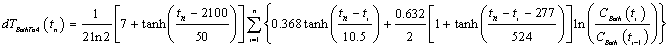# Global Warming Mathematics

## Introduction

Complicated climate models that require supercomputers to calculate them have yielded empirical functions that can be use by less-complicated computer models to study global warming. This article lists the equations used in such a less-complicated computer model of global warming. Using it enables one to easily test assumptions.

There are many nonlinear relations between variables in climate studies. That makes analytically solving equations for the interactions among the variables impossible; the calculations have to be done numerically. However, if one knows or can surmise some general features of the interaction, one can use nonlinear equations to connect the variables. For example, in this study the hyperbolic-tangent function is often used to approximate the nonlinear connection among variables.

For the details of and the references for the equations given here see http://www.roperld.com/science/GlobalWarmingprediction.htm .

## Coal Depletion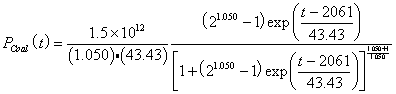short tons.## Crude-Oil Depletion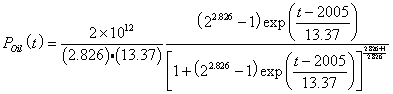barrels.## Natural-Gas Depletion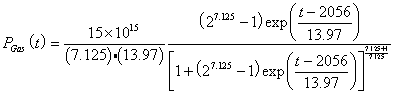1012 ft2.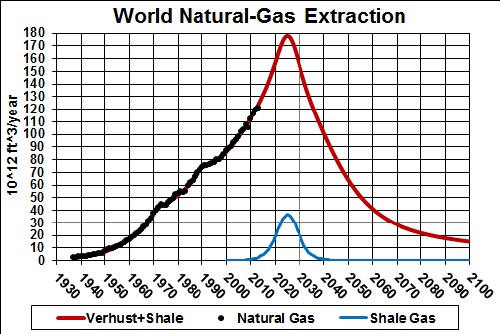## Carbon Emissions

The carbon emissions for fossil fuels are:,and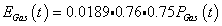,

assuming that 75% of each of the three fossil fuels is burned for fuel. Thus,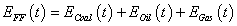.The assumption is made here that the fossil fuels are burned in the year they are extracted.

## Carbon-Dioxide Concentration in the Atmosphere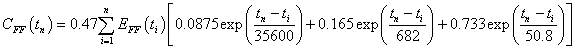for each year tn. The factor 0.47 is the canonical factor for converting carbon emissions (109 tonnes/year) to carbon-dioxide concentration (parts per million by volume, ppmv) in the atmosphere. The three exponentials represent the carbon-dioxide residence time. Below, the possibility of this factor increasing with concentration will be considered. The three exponentials yield the following CO2-concentration decay curve:Inserting the fossil-fuels emissions in the equation yields: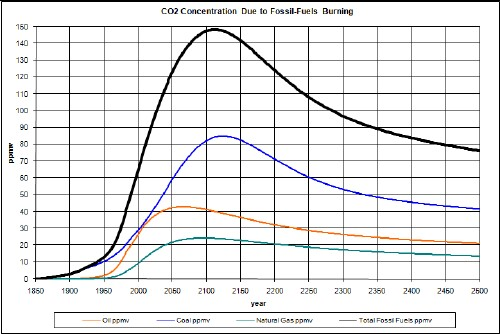Note that the carbon-dioxide peaks are several decades later than the fossil-fuels extraction peaks shown in the graphs above, due to the carbon-dioxide residence time.

Before fossil fuels were starting to be burned for energy, there was already a background concentration of carbon dioxide in the atmosphere of about 280 ppmv. Adding that background to the carbon-dioxide concentration due to burning fossil fuels yields:Note that the measured data are above the concentration due to fossil fuels, as it should be because there are other sources of carbon emissions, such as deforestation.

## Non-Fossil-Fuels Emissions

Possibly a good approximation for non-fossil-fuels (NFF) emissions is that its contribution to carbon-dioxide concentration is proportional to the fossil-fuels concentration. Rational for this approach is that the amount of deforestation, soil depletion, etc. is probably proportional to the amount of fossil fuels burned. Without fossil fuels these activities could not occur anywhere near the intensity that they do occur.

A linear fit yields a background concentration of about 292 ppmv, well above 280 ppmv, the known background concentration. It is assumed that this is due to pre-fossil-fuels emissions due to intensive agriculture and early industrialization. A hyperbolic tangent is used to represent the pre-fossil-fuels rise from c1700 to c1900: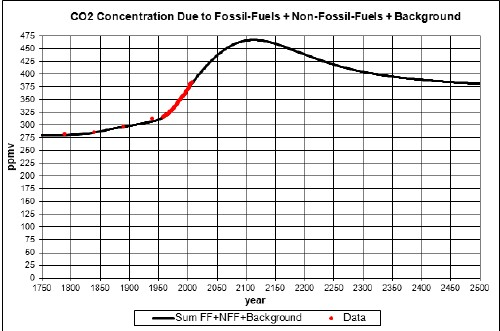## Temperature Change Due to Increasing Carbon Dioxide Concentration in the Atmosphere

I use a climate sensitivity of 3 degrees celsius for CO2 concentration doubling to calculate the approximate temperature change relative to year 1700, dT (in Celsius degrees), due to a change in CO2 concentration (in ppmv). The equation is: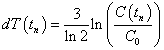for year tn relative to the concentration C0 for a reference year (1700 AD). The s=3 factor is the climate sensitivity that makes the climate sensitivity factor be for doubling of the CO2 concentration in the atmosphere, as it is defined. (Climate sensitivity is supposed to include the effects of fast feedback mechanisms, both positive and negative, in other variables caused by changing the CO2 concentration.)

An equivalent version of the equation is, where C(t0) = C0 .

Besides the climate sensitivity, one should allow for the fact that the ocean absorbs much of the solar energy impinging on the Earth and then slowly releases some of the heat energy to the atmosphere. There is a climate response function that represents the atmospheric temperature time delay after carbon is inserted into the atmosphere. The function is given by the data points in the following graph: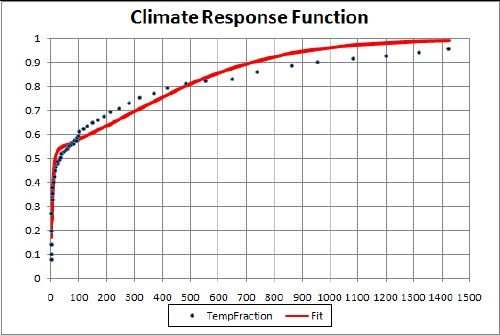The x-axis the number of years after the carbon dioxide is inserted into the atmosphere.

The two-hyperbolic-tangent fit is good because the data errors are large. The function is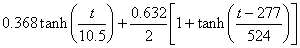A better fit could be obtained with three hyperbolic tangents, but the errors on the data do not justify it.

The resulting temperature change relative to 1700 due to fossil-fuels burning and non-fossil-fuels emissions is: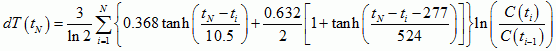An unknown amount of global dimming should be subtracted from this curve; it is probably only a few tenths of a degree or smaller and quite variable with time.

The peak would be higher if it were not for the time delay between carbon emission and the atmospheric temperature. The asymptotic temperature is the same for both calculations, even though the ocean keeps some of the energy, because the climate sensitivity accounts for that.

## Temperature Change for High Carbon-Dioxide Concentration

Suppose that the oceans and the vegetation reach a saturation point for high carbon-dioxide concentration such that the 0.47 factor doubles to 0.94 according to a hyperbolic tangent centered at 450 ppmv with a width of 25 ppmv. Then the concentration will be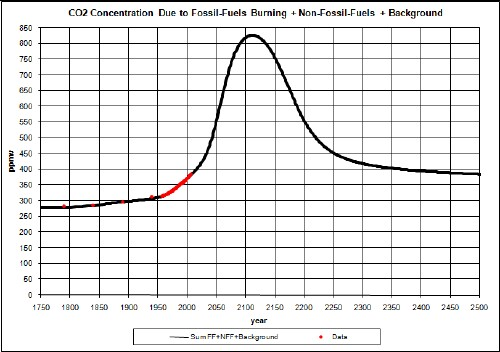The temperature would be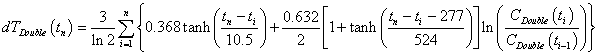## Carbon Release in the Arctic

Assume that global warming causes a large amount of carbon to be released into the atmosphere in the Arctic according to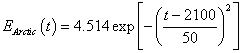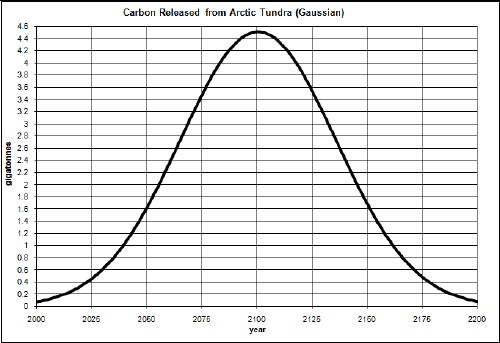The contribution to the carbon-dioxide concentration is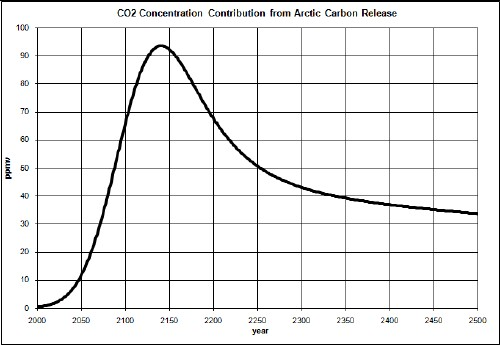So, the total carbon-dioxide concentration is now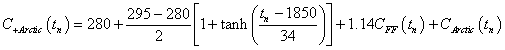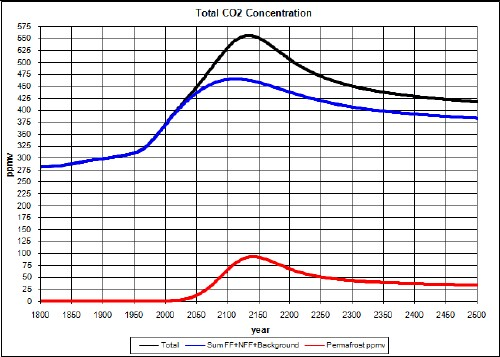The temperature would be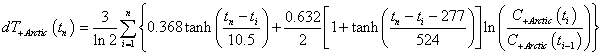## High Carbon-Dioxide Concentration Feedback, Arctic Carbon Release and Climate Sensitivity Increase (Worst Case)

Suppose both Arctic carbon release and high carbon-dioxide concentration feedback on the concentration occurs:The temperature would be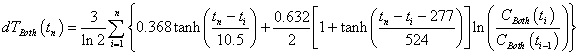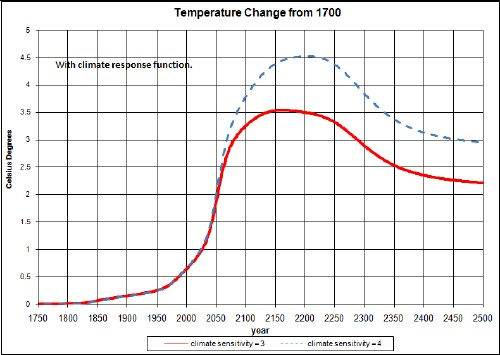The dashed curve is for a climate sensitivity changing from 3 to 4 by a hyperbolic-tangent function: